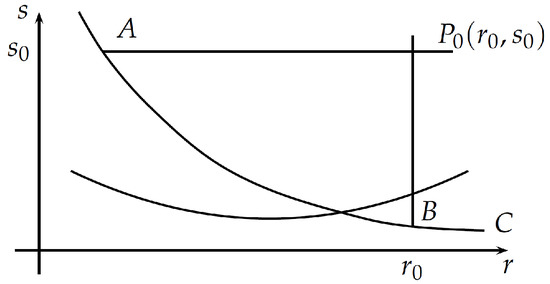Next Article in Journal
Statistical Analysis of Maximally Similar Sets in Ecological Research
Previous Article in Journal
Scaling Laws in the Fine-Scale Structure of Range Margins
Open AccessReview

# On the Riemann Function

byPeter J. Zeitsch
School of Mathematics and Statistics, University of Sydney, Sydney, NSW 2006, Australia
Mathematics 2018, 6(12), 316; https://doi.org/10.3390/math6120316
Received: 26 October 2018 / Revised: 6 December 2018 / Accepted: 6 December 2018 / Published: 10 December 2018
Riemann’s method is one of the definitive ways of solving Cauchy’s problem for a second order linear hyperbolic partial differential equation in two variables. The first review of Riemann’s method was published by E.T. Copson in 1958. This study extends that work. Firstly, three solution methods were overlooked in Copson’s original paper. Secondly, several new approaches for finding Riemann functions have been developed since 1958. Those techniques are included here and placed in the context of Copson’s original study. There are also numerous equivalences between Riemann functions that have not previously been identified in the literature. Those links are clarified here by showing that many known Riemann functions are often equivalent due to the governing equation admitting a symmetry algebra isomorphic to $S L ( 2 , R )$ . Alternatively, the equation admits a Lie-Bäcklund symmetry algebra. Combining the results from several methods, a new class of Riemann functions is then derived which admits no symmetries whatsoever. View Full-Text
Show FiguresFigure 1

MDPI and ACS Style

Zeitsch, P.J. On the Riemann Function. Mathematics 2018, 6, 316.